Back to my home page

# CMB B-modes from Faraday Rotation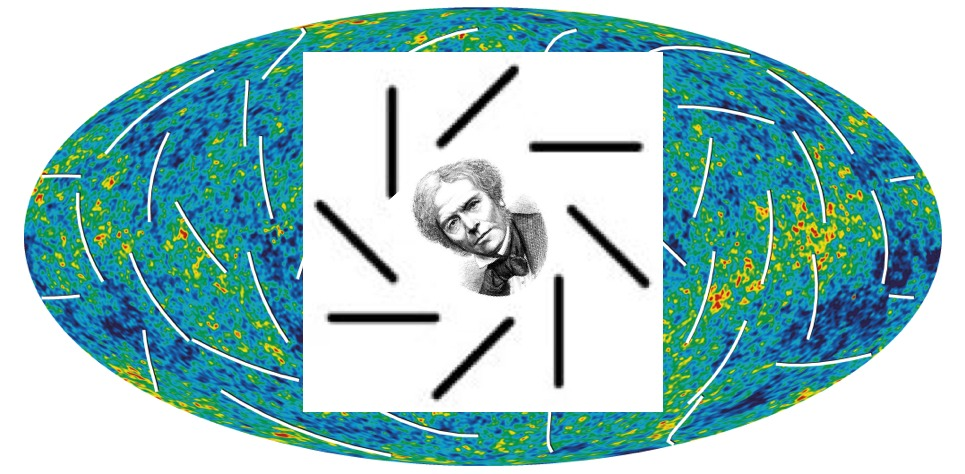# A quick and exact calculator of B-mode angular spectrum due to Faraday rotation by stochastic magnetic fields.

## Based on arXiv:1106.1438 by Levon Pogosian (SFU), Amit Yadav (IAS), Bess Ng (CWRU), and Tanmay Vachaspati (ASU)

Last updated on August 2, 2011

# B-modes from Faraday rotation

Magnetic fields generate B-mode polarization in the cosmic microwave background (CMB) due to Faraday rotation (FR) of the E-mode. Primordial fields, such as those generated in phase transitions in the early universe, can have significant power on scales much smaller than the thickness of the last scattering surface.

The CMB B-mode angular spectrum can be written in a form that separates the well-established physics of FR from the particular form of the magnetic field spectrum:

where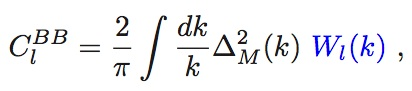(1)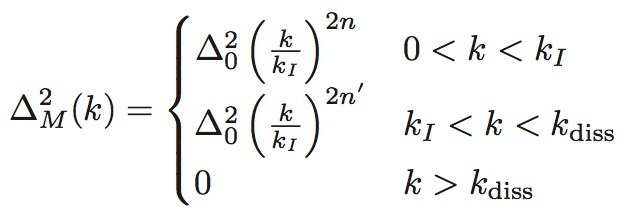,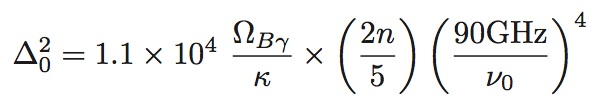(2)

contains the dependence on the frequency at which the CMB is observed, as well as the spectral form and normalization of the promordial magnetic spectrum. In the above,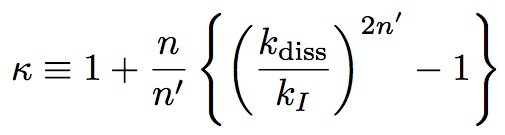,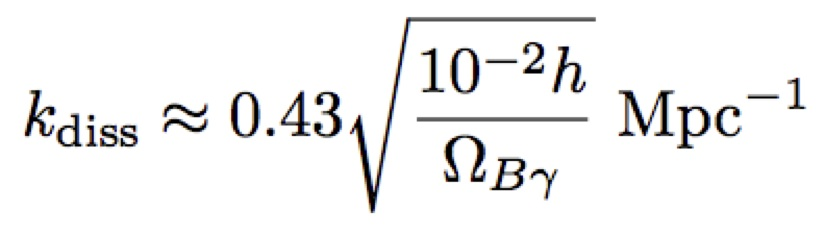.
The window functions Wl(k) are independent of the magnetic spectrum, and instead contain the information about the E-mode polarization spectrum and the background cosmology. Physically, a window function
Wl(k) prescribes how much power a given wavelength k of the magnetic field spectrum contributes to a given angular scale l of the B-mode polarization spectrum. We have calculated the window functions by numerically solving the full CMB radiative transport equation with FR taken into account. Once the window functions are calculated and stored, ClBB can be quickly calculated using (1) for a given set of parameters appearing in (2). For further details, and for the definitions of the parameters appearing in (2), please refer to the paper.

### Instructions for downloading and using the code

To acknowledge the use of the code, please cite our paper.

You can download the clbb-code.tar.gz file containing a short Fortran 77 code clbb.f and a data file window_functions.dat with functions Wl(k) stored on a grid in l and k. The range of l is from 2 to 10000, which sets the range of the output Cl. The range of k is from 0.0001 to 1 Mpc-1, which is sufficient for l<10000.

The code creates a file clbb.d where it saves the output as two columns. The first column is the multipole l (in the 2<l<10000 range), while the second column is the B-mode angular spectrum l(l+1)Cl/2pi in units of (muK)^2. The screenshot below illustrates the use of the code: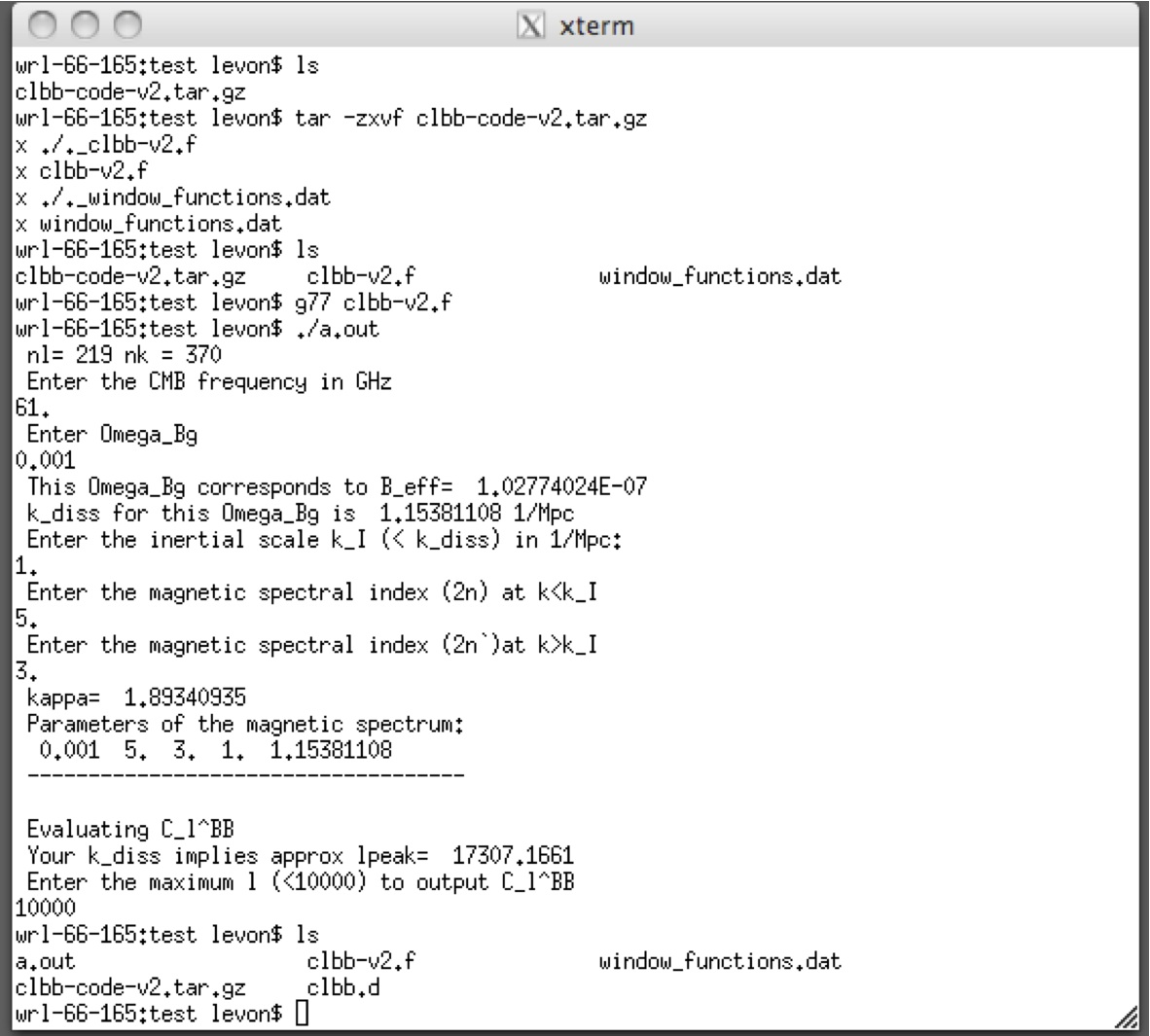When plotted, the data in clbb.d gives the red line in this plot, which is Fig. 4 in the paper: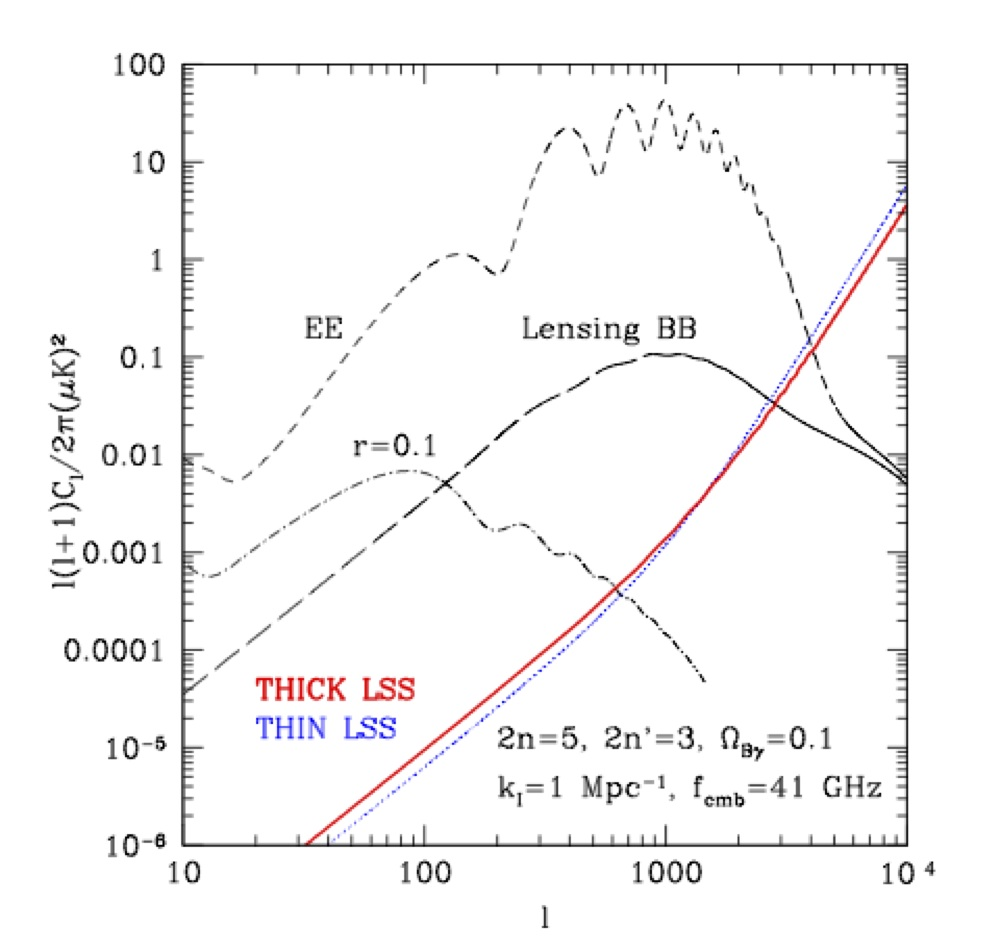With further questions e-mail levon<at>sfu.ca